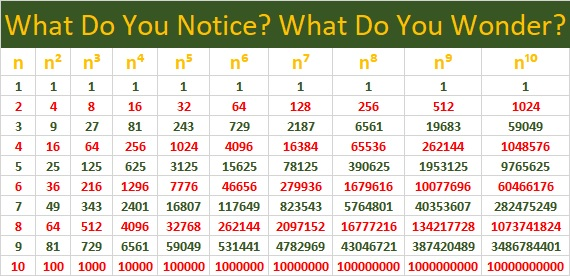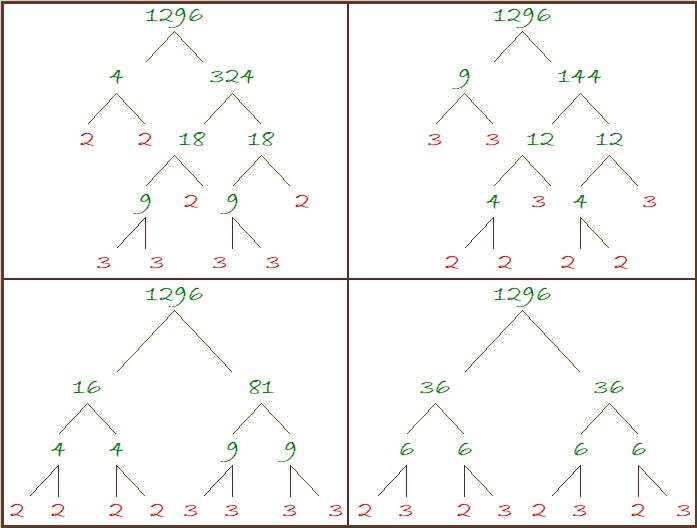# 1296 Last Digit Effort

If you always use a calculator to find the roots of perfect powers like 1296, then you will likely miss out on discovering the wonderful patterns those powers have. Look at the table below. What do you notice? What do you wonder?When I looked at it, I noticed several patterns and I wondered about a few things that I explored to see if they were true. However, I won’t tell you what I noticed or what conjectures I made until the end of this post, because I don’t want to spoil YOUR chance to discover those patterns yourself. I will tell you that there are MANY patterns in the table above and that most of my conjectures were true, but one of them turned out not to be.

Besides the patterns that you will have to discover for yourself, the table can be very useful: You can use the table to find several fractional powers of the numbers in the body of the table. For example, if you wanted to find out what is 1296^(7/4), then you could simply run your finger down the n⁷ column and the n⁴ column simultaneously until you reach 1296 on the n⁴ column (because 4 is the denominator of the fractional power). Your answer, 279936, will be in the n⁷ column on the same row as 1296.

However, if you wanted to find 1296^(3/2), you would either have to expand the table to include 36² and 36³, or you would have to use the fact that 3/2 = 6/4. Then you could use the process of the previous paragraph to see that
1296^(3/2) = 1296^(6/4) = 46656.

Now I’ll share some more facts about the number 1296:

• 1296 is a composite number, a perfect square, and a perfect fourth power.
• Prime factorization: 1296 = 2 × 2 × 2 × 2 × 3 × 3 × 3 × 3, which can be written 1296 = 2⁴ × 3⁴
• The exponents in the prime factorization are 4 and 4. Adding one to each and multiplying we get (4 + 1)(4 + 1) = 5 × 5 = 25. Therefore 1296 has exactly 25 factors.
• Factors of 1296: 1, 2, 3, 4, 6, 8, 9, 12, 16, 18, 24, 27, 36, 48, 54, 72, 81, 108, 144, 162, 216, 324, 432, 648, 1296
• Factor pairs: 1296 = 1 × 1296, 2 × 648, 3 × 432, 4 × 324, 6 × 216, 8 × 162, 9 × 144, 12 × 108, 16 × 81, 18 × 72, 24 × 54, 27 × 48, or 36 × 36
• Taking the factor pair with the largest square number factor, we get √1296 = (√36)(√36) = 36.1296 is the smallest number with exactly 25 factors.

There are MANY different factor trees you could make for 1296, but here are some made with squares:1296 is the 21st Friedman number because of its digits and 9²×16 = 1296. (The same digits are on both sides of that equation.)

Since 36 is a triangular number and 36² = 1296, we get this wonderful, powerful fact:
(1 + 2 + 3 + 4 + 5 + 6 + 7 + 8)² = 1³ + 2³ + 3³ + 4³ + 5³ + 6³ + 7³ + 8³ = 1296

1296 looks interesting and often rather square when it is written in several bases:
It’s1210000 in BASE 3,
10000 in BASE 6,
2420 in BASE 8,
1700 in BASE 9,
1296 in BASE 10
900 in BASE 12,
789 in BASE 13 (Ha, ha. Seven ate nine!),
484 in BASE 17,
400 in BASE 18,
169 in BASE 33,
144 in BASE 34,
121 in BASE 35, and
100 in BASE 36

Do you know the square roots in base 10 of all the numbers in bold? Indeed, 1296 is a fascinating square number!

Here are some things I noticed on the table of powers for numbers ending in . . .

• 1: The last digit is always 1
• 2: The pattern for the last digit is 2, 4, 8, 6, repeating
• 3: The pattern for the last digit is 3, 9, 7, 1, repeating
• 4: The pattern for the last digit is 4, 6, 4, 6, repeating
• 5: The last digit is always 5
• 6: The last digit is always 6
• 7: The pattern for the last digit is 7, 9, 3, 1, repeating (3’s pattern backward but starting with 7)
• 8: The pattern for the last digit is 8, 4, 2, 6, repeating (2’s pattern backward but starting with 8)
• 9: The pattern for the last digit is 9, 1, 9, 1, repeating
• 0: The last digit is always 0

For the squares:

• 1² and 9² both end in 1, and 1 + 9 = 10
• 2² and 8² both end in 4, and 2 + 8 = 10
• 3² and 7² both end in 9, and 3 + 7 = 10
• 4² and 6² both end in 6, and 4 + 6 = 10

For the cubes:

• 1³ ends in 1, and 9³ ends in 9
• 2³ ends in 8, and 8³ ends in 2; 2 + 8 = 10
• 3³ ends in 7, and 7³ ends in 3; 3 + 7 = 10
• 4³ ends in 4, and 6³ ends in 6

Other observations:

• No matter what n is, the last digit of n, n⁵, and n⁹ are always the same. Yes, that pattern continues with n¹³ and so forth.
• The number of digits for 10ᵃ is a+1, and the number of digits for 9ᵃ is a.

Dan Bach noticed something else.

I had never heard of Benford’s Law before, so I’ve learned something new here!  All of us can learn something new by observing patterns and listening to each other.

This site uses Akismet to reduce spam. Learn how your comment data is processed.